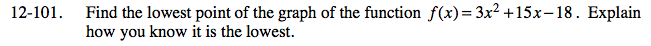### Home > CAAC > Chapter 12 > Lesson 12.4.4 > Problem12-101

12-101.The vertex is the lowest point of a parabola.

The x-coordinate of the vertex is halfway between the x-intercepts.

Substitute the x-coordinate of the vertex back into the equation to find the y-coordinate.

( −2.5, −36.75 )